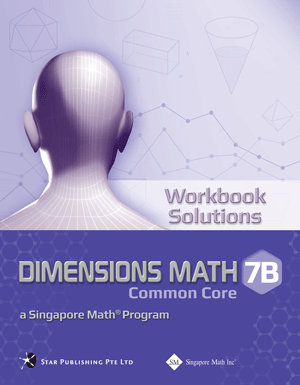# Singapore Math Dimensions Math Workbook Solutions 7B

\$ 16.50

Dimensions Math® Textbook 7B

Chapter 9: Number Patterns

9.1 Number Patterns and Sequences
9.2 General Term of a Sequence

A. General Term
B. Application of Number Patterns

In A Nutshell

Chapter 10: Coordinates and Linear Graphs

10.1 Cartesian Coordinate System
10.2 Linear Graphs
10.3 Slopes of Linear Graphs

A. Positive Slopes
B. Negative Slopes
C. Special Cases

In A Nutshell

Chapter 11: Inequalities

11.1 Solving Simple Inequalities

A. Idea of Inequalities
B. Solving an Inequality

11.2 More Properties of Inequalities
11.3 Simple Linear Inequalities
11.4 Application of Simple Inequalities

In A Nutshell

Chapter 12: Perimeters and Areas of Plane Figures

12.1 Perimeters and Areas of a Square, a Rectangle, and a Triangle
12.2 Circumference and Area of a Circle
12.3 Area of a Parallelogram
12.4 Area of a Trapezoid
12.5 Perimeters and Areas of Composite Plane Figures

In A Nutshell

Chapter 13: Volumes and Surface Areas of Solids

13.1 Volumes and Total Surface Areas of a Cube and a Cuboid

A. Nets of Cube, Cuboid, and Other Prisms
B. Cube
C. Cuboid

13.2 Volume and Total Surface Area of a Prism

A. Prism
B. Volume of Prism
C. Surface Area of Prism

13.3 Volumes and Surface Areas of Composite Solids

A. Conversion between Different Units
B. Composite Solids

In A Nutshell

Chapter 14: Proportions

14.1 Scale Drawing
14.2 Map Scale and Calculation of Area

A. Map Scale
B. Calculation of Area

14.3 Direct Proportion
14.4 Inverse Proportion

In A Nutshell

Chapter 15: Data Handling

15.1 Collection of Data
15.2 Dot Plots
15.3 Measure of Center: Mean

A. Measure of Center
B. Mean
C. Using Mean to Compare Two Populations
D. Variation from the Mean

15.4 Measure of Center: Median
15.5 Mode

A. Definition of Mode
B. Comparison between Mean, Median, and Mode

In A Nutshell

Chapter 16: Probability of Simple Events

16.1 Set Notation

A. Sets
B. Equal Sets
C. Subsets
D. Universal Set and Empty Set
E. Complement of a Set

16.2 The Meaning of Probability

A. Introduction
B. Terms and Definitions

16.3 Sample Space

A. Sample Space
B. Basic Properties of Probabilities

In A Nutshell

Chapter 17: Probability of Combined Events

17.1 Probability of Simple Combined Events

A. Possibility Diagram
B. Tree Diagram

17.2 The Meaning of Probability
17.3 Independent Events
17.4 Further Probabilities

In A Nutshell

Dimensions Math® G6-8 Textbooks are designed for middle school students. Developed in collaboration between Star Publishing and Singapore Math Inc®, this series follows the Singapore Mathematics Framework and covers the topics in the Common Core State Standards.

The emphasis of this series is on empowering the students to learn mathematics effectively and independently. Depending on the topics covered, different approaches are adopted for the presentation of concepts to facilitate easy understanding by students to internalize concepts and instill in them an interest to explore the topics further.

Each book includes appropriate examples, class activities and diagrams to understand the concepts and apply them. IT skills and values are incorporated as appropriate.

Some features of this series include the following:

1. Class activities which allow the student to learn mathematics through discovery. Some of the class activities make use of Geometer’s Sketchpad (available at keypress.com).
2. Worked examples are followed by a similar question (Try It!) so that students can check if they have understood the concepts presented earlier.
3. An exercise for each lesson including questions in the following sequence:
• Basic Practice (simple questions involving a direct application of the concepts)
• Further Practice (more challenging questions on direct application)
• Maths@Work (questions that apply mathematical concepts to real-life situations)
• Brainworks (questions involving higher order thinking or an open-ended approach to problems)
4. Each chapter is followed by a review exercise; the Extend Your Learning Curve activity encourages students to explore mathematical concepts further or apply mathematics in real-life situations, with some questions requiring sentence or paragraph answers to encourage students to reflect on their learning experiences.
5. The right margins contain remarks on important information, concepts or definitions covered earlier related to the current material to help students recall them, discussion questions, interesting puzzles or facts that are related to mathematics, and some website references.

The answer key at the back of the book provides answers to the Try It! and the problems in the exercises for the Basic PracticeFurther Practice, and Maths@Work questions. It does not include answers to the class activities or the Extend Your Learning Curve activities.